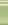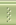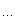# MemoryGarden's BlogC++博客首页新随笔联系聚合管理#include <stdio.h>
#include
<string.h>
const int _ = 0x7fffffff;
struct e{

int u, v, w;
e(
int a, int b, int c){u = a; v = b; w = c;}
e(){}
};
int n, m, el, d;e edge;int path;
bool relax(int u, int v, int w){//松弛操作
if(d[u] != _ && d[v] > d[u] + w){
path[v]
= u;
d[v]
= d[u] + w;

return true;
}
return false;
}
bool bellman(){

int i, j, u, v, w;bool ok;

for(i = 0; i < n - 1; i++){//点 - 1此松弛操作
ok = true;

for(j = 0; j < el; j++){
u
= edge[j].u;v = edge[j].v;w = edge[j].w;

if(relax(u, v, w))ok = false;
}
if(ok)break;//若此次更新没有对边的松弛，则可以跳出了
}

for(i = 0; i < el; i++){
u
= edge[i].u; v = edge[i].v;w = edge[i].w;

if(d[u] != _ && d[v] > d[u] + w)return false;//验证是否有负环
}

return true;
}

void init(){

int i;

for(i = 0; i < n; i++)d[i] = _;//初始化最短路径估计值，以及路径
memset(path, -1sizeof(path));
d[
0= 0;el = 0;//源点的估计值为0
}

int main(){
freopen(
"bellman.in""r", stdin);
freopen(
"bellman.out""w", stdout);

int i, j, u, v, w;

while(scanf("%d%d"&n, &m) != -1){
init();

for(i = 0; i < m; i++){
scanf(
"%d%d%d"&u, &v, &w);
edge[el].u
= u;edge[el].v = v;edge[el++].w = w;
}i
= bellman();

if(i){

for(i = 0; i < n; i++){
printf(
"shortest value from 0 to point %d = %d\n", i, d[i]);
}
}
else{
puts(
"..");
}
}

return 0;
}

posted on 2009-10-06 23:49 memorygarden 阅读(147) 评论(0)  编辑 收藏 引用 所属分类: 图论